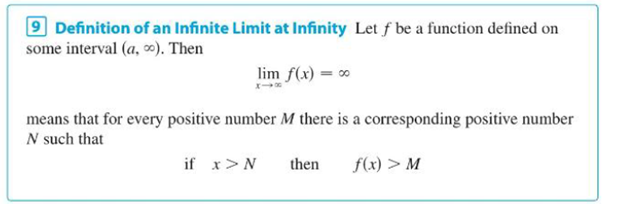Chapter 2.6, Problem 74E

Chapter
Section
Textbook Problem

For the limit lim x → ∞ x ln x = ∞ illustrate Definition 9 by finding a value of N that corresponds to M = 100.Definition 9To determine

To find: The value of N for the limit limxxlnx= that correspond to M=100.

Explanation

Definition: Let f be a function defined on some interval (a,). Then limxf(x)= means that for every positive number M there is a corresponding positive number N such that if x>N then f(x)>M.

Proof:

Let f(x)=xlnx and M=100.

By the definition,

f(x)>Mxlnx>100

Squaring on both sides.

xlnx>(100)2xlnx>10,000xlnx10,000>1xlnx>10,000

Differentiate on both sides.

[x×1x+(1)×lnx]>0[1+lnx]>0

Take exponential on both sides

Still sussing out bartleby?

Check out a sample textbook solution.

See a sample solution

The Solution to Your Study Problems

Bartleby provides explanations to thousands of textbook problems written by our experts, many with advanced degrees!

Get Started

In Exercises 6372, evaluate the expression. 68. 1+22

Applied Calculus for the Managerial, Life, and Social Sciences: A Brief Approach

Solve the equations in Exercises 126. (x+1)2(x+2)3(x+1)3(x+2)2(x+2)6=0

Finite Mathematics and Applied Calculus (MindTap Course List)

Elimination of the parameter in x = 2t3/2, y = t2/3 gives: x4 = 16y9 16x4 = y4 x3 = 8y4 8x3 = y4

Study Guide for Stewart's Single Variable Calculus: Early Transcendentals, 8th